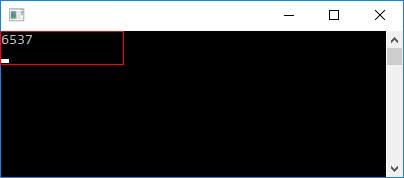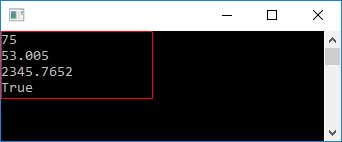# CS Type Conversion

Type conversions is a process by which one type is converted to another type. We can not only convert data types with C # conversion techniques, but we also convert object types. The conversion type in C# can be either implicit or explicit.

When one data type is converted automatic into another data type, it is known as a conversion implicitly. Due to implicit conversions, there is no data loss.

Explicit conversion is a forced conversion and data loss may occur. Users use the predefined functions to make these conversions explicitly. Cast operators are necessary for explicit conversions. Type conversions occur mainly by passing arguments on an arithmetic function or mix mode, etc.

Example:

``````
using System;

namespace TypeConversionApplication {
class ExplicitConversion {
static void Main(string[] args) {
double d = 6537.47;
int i;

// cast double to int.
i = (int)d;
Console.WriteLine(i);
}
}

``````

Output:The following integrated conversion methods are provided with C#.

 Methods Decriptions ToBoolean() Converts a type to a Boolean value, where possible. ToByte() Converts a type to a byte. ToChar() Converts a type to a single Unicode character, where possible. ToDateTime() Converts a type (integer or string type) to date-time structures. ToDecimal() Converts a floating point or integer type to a decimal type. ToDouble() Converts a type to a double type. ToInt16() Converts a type to a 16-bit integer. ToInt32() Converts a type to a 32-bit integer. ToInt64() Converts a type to a 64-bit integer. ToSbyte() Converts a type to a signed byte type. ToSingle() Converts a type to a small floating point number. ToString() Converts a type to a string. ToType() Converts a type to a specified type. ToUInt16() Converts a type to an unsigned int type. ToUInt32() Converts a type to an unsigned long type. ToUInt64() Converts a type to an unsigned big integer.

Example:

``````
using System;

namespace TypeConversionApplication {
class StringConversion {
static void Main(string[] args) {
int i = 75;                          //integer
float f = 53.005f;              // Float
double d = 2345.7652;    // double
bool b = true;                   //boolen

Console.WriteLine(i.ToString());
Console.WriteLine(f.ToString());
Console.WriteLine(d.ToString());
Console.WriteLine(b.ToString());

}
}
}

``````

Output: